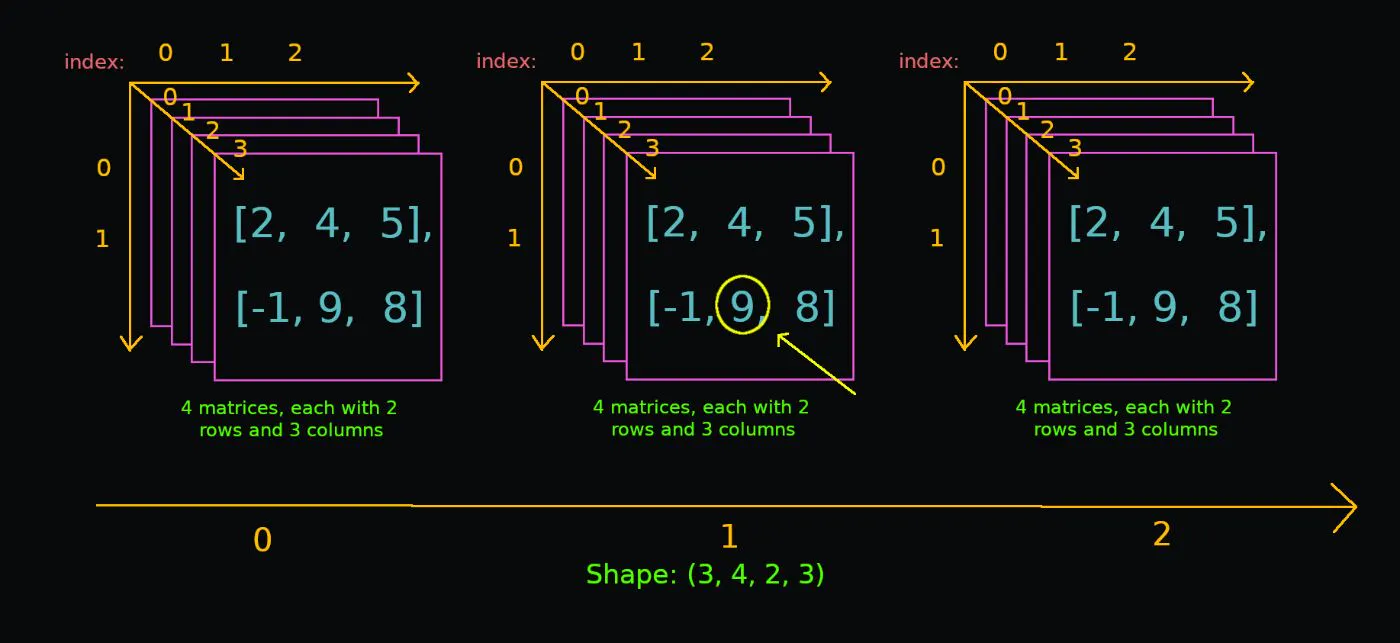A Quick Introduction to Python Numpy for Beginnersby@biraj21

# A Quick Introduction to Python Numpy for BeginnersNumPy is a Python library mainly used to work with arrays. An array is a collection of items that are stored next to each other in memory. The calculations in NumPy are done by the parts that are written in C, which makes them extremely fast compared to normal Python code. We can create a NumPy array by using the numpy module's `array()` function. The type of our `arr` variable is `numpy.ndarray, which stands for N-dimensional array.### @biraj21

Biraj